# Test: Reaction Mechanism Level - 5

## 30 Questions MCQ Test Organic Chemistry | Test: Reaction Mechanism Level - 5

Description
Attempt Test: Reaction Mechanism Level - 5 | 30 questions in 60 minutes | Mock test for Chemistry preparation | Free important questions MCQ to study Organic Chemistry for Chemistry Exam | Download free PDF with solutions
QUESTION: 1

Solution:
QUESTION: 2

Solution:
QUESTION: 3

### In the cyclisation reaction given below, the most probable product formed is: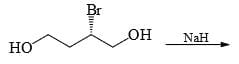Solution:
QUESTION: 4

The major product formed in the reaction given below is

: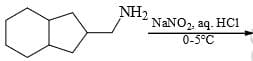Solution:
QUESTION: 5

The major product formed in the following reaction is: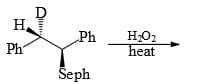Solution:
QUESTION: 6

The correct order of the solvolysis for the following chlorides in acetic acid is: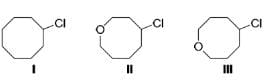Solution:
QUESTION: 7

The correct order of reactivity of p-halonitrobenzens in the following reaction is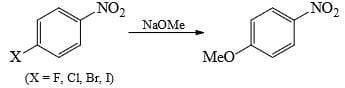Solution:
QUESTION: 8

Solvolysis of the optically active compound X gives, mainly: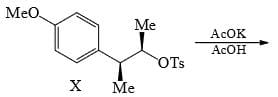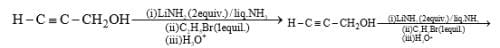Solution:
QUESTION: 9

The major product obtained in the following reaction, is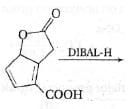Solution: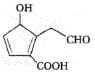Explanation:
DIBAL-H (Di-isobutyl aluminium hydride) is a reducing agent with the preparation of aldehydes. Using DIBAL - H, Lactones are reduced directly to aldehydes.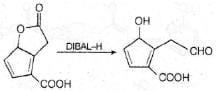QUESTION: 10

The major product formed in the following reaction is: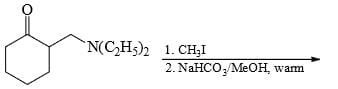Solution:
QUESTION: 11

Increasing order of stability of following carbocations (give least stable first)?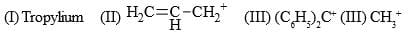Solution:
QUESTION: 12

What is the nucleophilicity order for SN2 reaction: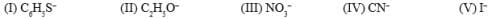Solution:
QUESTION: 13

Select order of effectiveness of Lewis acid catalyst in Friedel-Crafts reaction:

Solution:
QUESTION: 14

For the reaction between alkyl halide and OH- increase in solvent polarity generally

Solution:
QUESTION: 15

In an SN2 reaction there is:

Solution:
QUESTION: 16

Reactive intermediate formed in the following reaction is: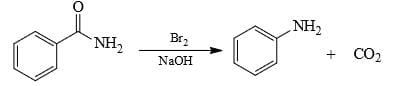Solution: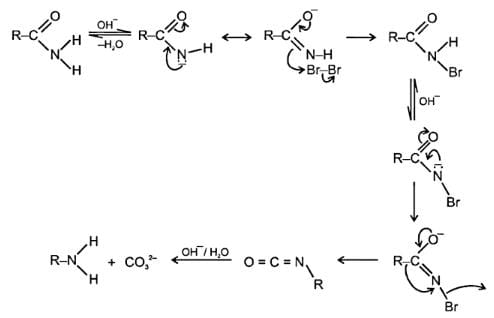QUESTION: 17

Cannizzaro reaction is not given by:

Solution:
QUESTION: 18

An SN2 reaction at an asymmetric carbon atom of a dextro alkyl halide always gives a:

Solution:
QUESTION: 19

Reaction of ethyne with HCN in presence of Ba (CN)2 is an example of:

Solution:
QUESTION: 20

Consider the following carbocations is most stable:

Solution:
QUESTION: 21

When 2-chloro-2-methylbutane is refluxed with alcoholic KOH, the main product obtained is:

Solution:
QUESTION: 22

Using given codes, arrange the following compounds in decreasing order of the rate of solvolysis by SN1 mechanism: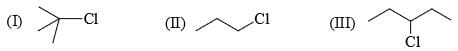Solution:
QUESTION: 23

1, 3-Dichloropropane one reaction with Zn and NaI gives:

Solution:
QUESTION: 24

Which is the final main product of the following reaction of trans-1,2-dibromocyclohexane?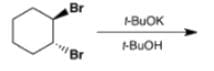Solution:

c)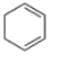Elimination reactions by the E2 mechanism are facilitated when the nucleofuge and H on adjacent C atoms can achieve an antiperiplanar relationship. This is only possible in chair trans-1,2-dibromocyclohexane when both Br atoms are axial. The Br at C1 can then be eliminated with a trans axial H on C6 from this (unfavourable) conformation, and the Br at C2 can be eliminated with a trans axial H on C3. Either way, the product is 3-bromocyclohexene which can then undergo a further E2 elimination to give cyclohexa-1,3-diene.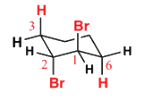QUESTION: 25

The intermediate in the reaction of m-bromoanisole with sodamide in liquid amnonia has:

Solution:
QUESTION: 26

Which one is least stable carbanion:

Solution:
QUESTION: 27

Which of the alkyl halides undergoes most readily for nucleophilic substitution reaction:

Solution:
QUESTION: 28

The reaction of ethanolic KOH on 1, 1-dichloropropane gives:

Solution:
QUESTION: 29

Which reactive intermediate is believed to be part of the reaction shown: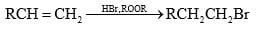Solution:
QUESTION: 30

In this transformation,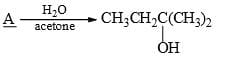What is the best structure for A?

Solution:Use Code STAYHOME200 and get INR 200 additional OFF Use Coupon Code